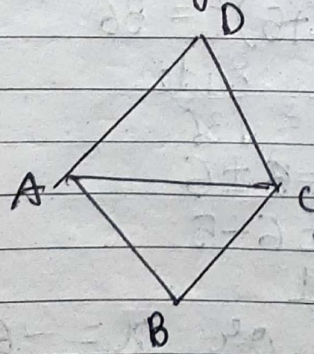In a rhombus ABCD, AC=AB. State the measure of ∠D and ∠BAD respectively.

# In a rhombus . State the measure of $\angle D$ and $\angle \mathit{BAD}$ respectively.

1. A
2. B
3. C
4. D

Fill Out the Form for Expert Academic Guidance!l

+91

Live ClassesBooksTest SeriesSelf Learning

Verify OTP Code (required)

### Solution:

Let the figure be -Given: $\mathit{AC}=\mathit{AB}$
As we know, all sides and diagonals of a rhombus is equal -
So,
$\mathit{AB}=\mathit{BC}=\mathit{CD}=\mathit{DA}=\mathit{AC}$
Here, $\mathit{AC}$ is diagonal of rhombus,
Now,
In $\mathit{\Delta ACD}$ and $\mathit{\Delta ABC}$ is equilateral $\Delta$ because here all sides are equal.
So, in $\mathit{\Delta ACD}$ -
$\mathit{AC}=\mathit{CD}=\mathit{DA}$
∴ Each angle of equilateral $\Delta$ is $60°$
As we know, rhombus is also parallelogram and sum of adjacent angles of parallelogram is $180°$.
$\angle D+\angle A=180°$
$60°+\angle A=180°$
$\angle A=180°-60°$
$\angle A=120°$
$\angle \mathit{BAD}=120°$

## Related content

 Area of Square Area of Isosceles Triangle Pythagoras Theorem Triangle Formula Perimeter of Triangle Formula Area Formulae Volume of Cone Formula Matrices and Determinants_mathematics Critical Points Solved Examples Type of relations_mathematics+91

Live ClassesBooksTest SeriesSelf Learning

Verify OTP Code (required)